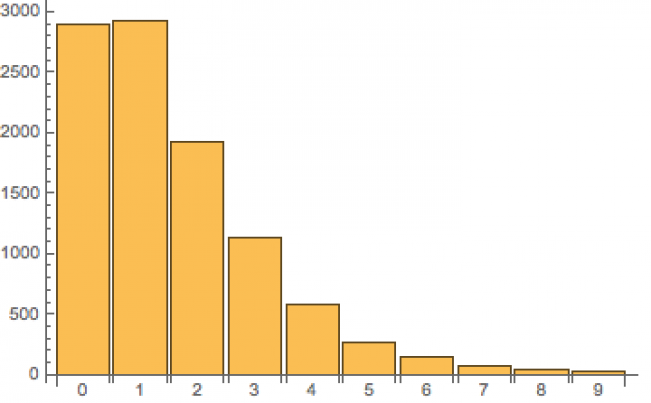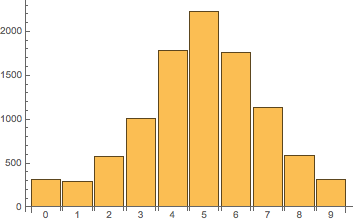# 略微加速

## 略速 - 互联网笔记

2020-05-08 10:17:17 leiting (3145阅读)

JavaScript 开发中有时会遇到要将一个数组随机排序(shuffle)的需求，一个常见的写法是这样：

```function shuffle(arr) {

arr.sort(function () {

return Math.random() - 0.5;

});

}```

```function shuffle(arr) {

arr.sort(() => Math.random() - 0.5);

}```

```let n = 10000;

let count = (new Array(10)).fill(0);

for (let i = 0; i < n; i ++) {

let arr = ['a', 'b', 'c', 'd', 'e', 'f', 'g', 'h', 'i', 'j'];

arr.sort(() => Math.random() - 0.5);

count[arr.indexOf('a')]++;

}

console.log(count);``````let a = ['a', 'b', 'c', 'd', 'e', 'f', 'g', 'h', 'i', 'j', 'k']; // 长度为11

let n = 10000;

let count = (new Array(a.length)).fill(0);

for (let i = 0; i < n; i ++) {

let arr = [].concat(a);

arr.sort(() => Math.random() - 0.5);

count[arr.indexOf('a')]++;

}

console.log(count);```

sort_03

Calling comparefn(a,b) always returns the same value v when given a specific pair of values a and b as its two arguments.

```let new_i = {

v: i,

r: Math.random()

};```

`arr.sort((a, b) => a.r - b.r);`

```function shuffle(arr) {

let new_arr = arr.map(i => ({v: i, r: Math.random()}));

new_arr.sort((a, b) => a.r - b.r);

arr.splice(0, arr.length, ...new_arr.map(i => i.v));

}

let a = ['a', 'b', 'c', 'd', 'e', 'f', 'g', 'h', 'i', 'j'];

let n = 10000;

let count = (new Array(a.length)).fill(0);

for (let i = 0; i < n; i ++) {

shuffle(a);

count[a.indexOf('a')]++;

}

console.log(count);```

```-- To shuffle an array a of n elements (indices 0..n-1):

for i from n−1 downto 1 do

j ← random integer such that 0 ≤ j ≤ i

exchange a[j] and a[i]```

```function shuffle(arr) {

let i = arr.length;

while (i) {

let j = Math.floor(Math.random() * i--);

[arr[j], arr[i]] = [arr[i], arr[j]];

}

}```

```function shuffle(arr) {

var i = arr.length, t, j;

while (i) {

j = Math.floor(Math.random() * i--);

t = arr[i];

arr[i] = arr[j];

arr[j] = t;

}

}```